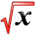# Increasing/Decreasing/ConcavityThis article is part of the MathHelp Tutoring Wiki

## Definition

Any function $f$is

• increasing at $x$if $f'(x)>0$• decreasing at $x$if $f'(x)<0$• concave up at $x$if $f''(x)>0$• concave down at $x$if $f''(x)<0$Critical point is the point where $f'(x)=0$. A critical point is

• maxima where $f''(x)<0$• minima where $f''(x)>0$• inflection point if $f''(x)=0$and $f''(x)$does change sign around $x$## Critical Points

Critical Points are points where $f'(x)=0$Notice that at critical points the function is neither increasing not decreasing.

You can comment on increasing/decreasing on either side of critical points.